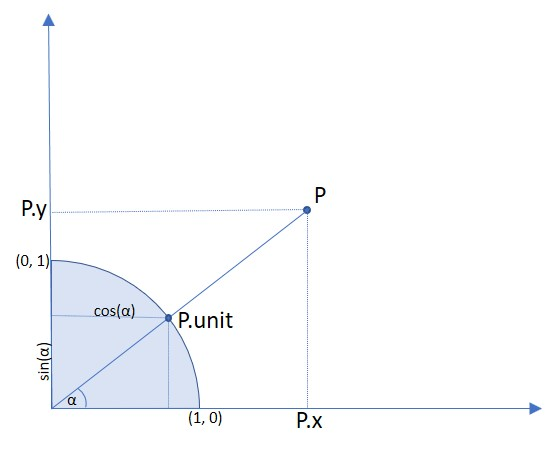# Point#

Point represents a point in the plane, defined by its x and y coordinates.

Attribute / Method

Description

`Point.distance_to()`

calculate distance to point or rect

`Point.norm()`

the Euclidean norm

`Point.transform()`

transform point with a matrix

`Point.abs_unit`

same as unit, but positive coordinates

`Point.unit`

point coordinates divided by abs(point)

`Point.x`

the X-coordinate

`Point.y`

the Y-coordinate

Class API

class Point#
__init__(self)#
__init__(self, x, y)#
__init__(self, point)#
__init__(self, sequence)#

Without parameters, Point(0, 0) will be created.

With another point specified, a new copy will be created, “sequence” is a Python sequence of 2 numbers (see Using Python Sequences as Arguments in PyMuPDF).

Parameters:
• x (float) – x coordinate of the point

• y (float) – y coordinate of the point

distance_to(x[, unit])#

Calculate the distance to x, which may be `point_like` or `rect_like`. The distance is given in units of either pixels (default), inches, centimeters or millimeters.

Parameters:
• x (point_like,rect_like) – to which to compute the distance.

• unit (str) – the unit to be measured in. One of “px”, “in”, “cm”, “mm”.

Return type:

float

Returns:

the distance to x. If this is `rect_like`, then the distance

• is the length of the shortest line connecting to one of the rectangle sides

• is calculated to the finite version of it

• is zero if it contains the point

norm()#
• New in version 1.16.0

Return the Euclidean norm (the length) of the point as a vector. Equals result of function abs().

transform(m)#

Apply a matrix to the point and replace it with the result.

Parameters:

m (matrix_like) – The matrix to be applied.

Return type:

Point

unit#

Result of dividing each coordinate by norm(point), the distance of the point to (0,0). This is a vector of length 1 pointing in the same direction as the point does. Its x, resp. y values are equal to the cosine, resp. sine of the angle this vector (and the point itself) has with the x axis.Type:

Point

abs_unit#

Same as `unit` above, replacing the coordinates with their absolute values.

Type:

Point

x#

The x coordinate

Type:

float

y#

The y coordinate

Type:

float

Note

This software is provided AS-IS with no warranty, either express or implied. This software is distributed under license and may not be copied, modified or distributed except as expressly authorized under the terms of that license. Refer to licensing information at artifex.com or contact Artifex Software Inc., 39 Mesa Street, Suite 108A, San Francisco CA 94129, United States for further information.